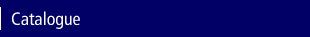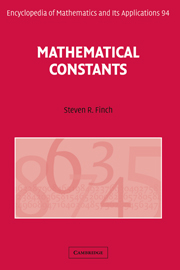Home > Catalogue > Mathematical ConstantsSearch this book

## Details

• 62 b/w illus. 9 tables
• Page extent: 624 pages
• Size: 234 x 156 mm
• Weight: 0.99 kg

## Library of Congress

• Dewey number: 513
• Dewey version: 21
• LC Classification: QA41 .F54 2003
• Mathematical constants

Library of Congress Record

### Hardback

(ISBN-13: 9780521818056 | ISBN-10: 0521818052)

• Also available in Adobe eBook
• ### For price and ordering options, inspection copy requests, and reading lists please select:

Please note local prices may apply

Famous mathematical constants include the ratio of circular circumference to diameter, pi=3.14 …, and the natural logarithmic base, e=2.178 … Students and professionals usually can name at most a few others, but there are many more buried in the literature and awaiting discovery. How do such constants arise, and why are they important? Here Steven Finch provides 136 essays, each devoted to a mathematical constant or a class of constants, from the well known to the highly exotic. Topics covered include the statistics of continued fractions, chaos in nonlinear systems, prime numbers, sum-free sets, isoperimetric problems, approximation theory, self-avoiding walks and the Ising model (from statistical physics), binary and digital search trees (from theoretical computer science), the Prouhet-Thue-Morse sequence, complex analysis, geometric probability and the traveling salesman problem. This book will be helpful both to readers seeking information about a specific constant, and to readers who desire a panoramic view of all constants coming from a particular field, for example combinatorial enumeration or geometric optimization. Unsolved problems appear virtually everywhere as well. This is an outstanding scholarly attempt to bring together all significant mathematical constants in one place.

• Broad spectrum of mathematics is covered, even statistical physics and theoretical computer science • The mathematical story of each constant is given clearly and concisely, with formulas, interrelationships, and applications • Full bibliographies are provided, with pointers to the most recent, cutting-edge research

### Contents

Preface; Notation; 1. Well-known constants; 2. Constants associated with number theory; 3. Constants associated with analytic inequalities; 4. Constants associated with the approximation of functions; 5. Constants associated with enumerating discrete structures; 6. Constants associated with functional iteration; 7. Constants associated with complex analysis; 8. Constants associated with geometry; Table; Index.

### Prize Winner

Lester R. Ford Award for Expository Excellence 2005 - Winner

### Reviews

'I think all mathematicians should own this book … there are sections where the author conveys admirably his excitement over some unexpected and beautiful sequence of ideas. I pay the author the earnest compliment of stating that nearly every page in the book is an adventure …'. The Mathematical Intelligencer

'… very accessible … The author's clear and engaging style makes the book a pleasure to read …'. MAA Online

'This is a very useful book … should be in each mathematical library.' Monatshefte für Mathematik

'This is a very useful book … The emphasis is on the mathematical origin of the constants and their interrelationships. The aim is always to be clear and complete, to motivate why a particular constant or idea is important, and to indicate exactly where in the literature one should look for rigorous proofs and further elaboration. This book should be in each mathematical library.' Internationale Mathematische Nachrichten

'Enormous fun, and you don't even have to follow any difficult arguments; a treasure-trove for the mathematical beachcomber.' The Mathematical Gazette

'This is a very useful book. The aim is always to be clear and complete, to motivate why a particular constant of idea is important, and to indicate exactly where in the literature one should look for rigorous proofs and further elaboration. This book should be in each mathematical library.' Internationale Mathematische Nachrichten

'This excellent book contains 136 'stories', each devoted to a mathematical constant or a class of constants, from the well known and famous … to the highly exotic and obscure ones. … The book is very useful and interesting for all mathematicians looking for information about a lot of specific constants, because it brings together all significant mathematical constants in one place.' Acta Scientiarum Mathematicarum